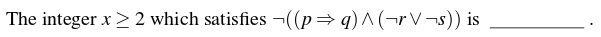Skip to content
Related Articles
GATE | GATE-CS-2016 (Set 1) | Question 11
• Last Updated : 12 Feb, 2016

Let p, q, r, s represent the following propositions.

```p: {8, 9, 10, 11, 12}
q: x is a composite number
r: x is a perfect square
s: x is a prime number```Note : This question was asked as Numerical Answer Type.
(A) 8
(B) 9
(C) 11
(D) 12

Answer: (C)

Explanation: (p ⇒ q) will give {8, 9, 10, 12}
¬r will give {8, 10, 11, 12}
¬s will give {8, 9, 10, 12}
(¬r ∨ ¬s) will give {8, 9, 10, 11, 12}
(p ⇒ q) ∧ (¬r ∨ ¬s) will give {8, 9, 10, 12}
¬((p ⇒ q) ∧ (¬r ∨ ¬s)) will give 11.

Thus, C is the correct option.

Quiz of this Question

Attention reader! Don’t stop learning now. Learn all GATE CS concepts with Free Live Classes on our youtube channel.

My Personal Notes arrow_drop_up